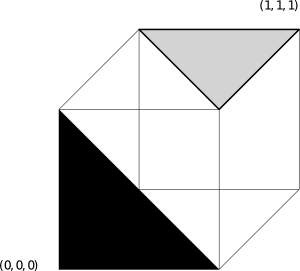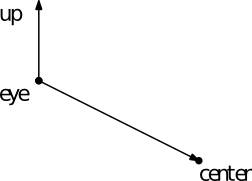UNB/ CS/ David Bremner/ teaching/ cs4735/ milestones/ Class test 1

## Important information

• the test will be Friday October 21 in class.

## Practice questions

### 1. transforms

#### 2D

Give an example in 2D that shows that scaling and rotations are not always commutative.

#### 3DGive a transformation matrix that maps the black triangle to the gray one. You may leave your answer as a product of matrices, with rotations given symbolically as e.g. Rz(45) meaning rotate CCW 45 degrees about the z axis.

### 2. Camera CoordinatesExplain how to compute the view matrix give the eye position, the up vector, and the point center being looked at.

### 3. Projection matrices

#### Example projection/depth transform matrix

Consider the matrix

P =

1 0 0 0
0 1 0 0
0 0 3 -2
0 0 1 0

1. Describe the effect of the matrix, both geometrically and in the context of a rendering pipeline.

2. Describe the effect of applying this transformation twice.

#### Perspective projection matrix

Give a matrix that implements the following perspective transformation (D is the distance to the near clipping plane). Explain your answer.

``````   [x,y,z,w]ᵀ → [Dx/z, Dy/z, D]
``````

### 4. Clipping

Give robust inequlities to clip a homogeneous point `[x,y,z,w]ᵀ` against the standard cube `[-1,1]×[-1,1]×[-1,1]`. Are there any special cases? If so, explain.